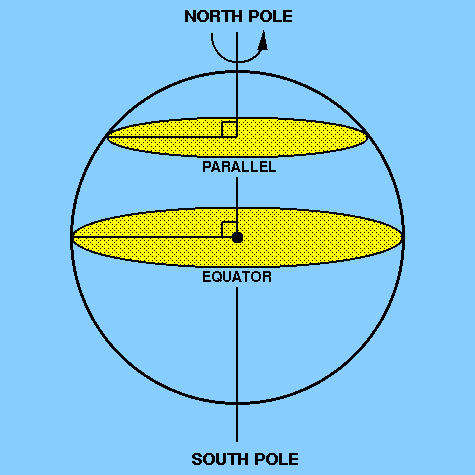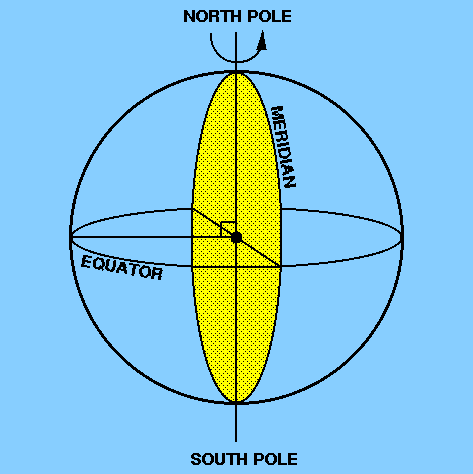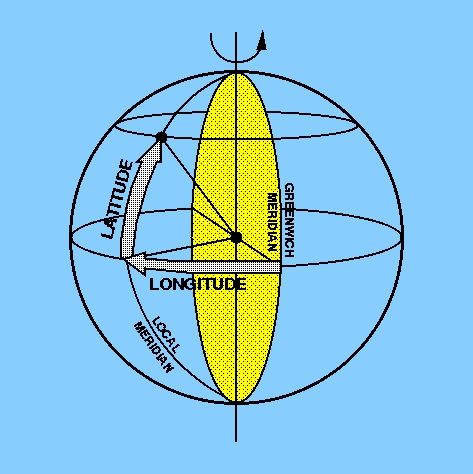To see how some of the spherical geometry described above can be put to good use, let us look at an everyday example - the measurement of position and distance on the surface of the Earth. The rotation of the Earth on its axis presents us with an obvious means of defining a coordinate system for the surface of the Earth. The two points where the rotation axis meets the surface of the Earth are known as the north pole and the south pole and the great circle perpendicular to the rotation axis and lying half-way between the poles is known as the equator. Great circles which pass through the two poles are known as meridians and small circles which lie parallel to the equator are known as parallels. Figure 5 and Figure 6 illustrate the above definitions.

 figure 5: the north pole, the south pole, the equator and a parallelfigure 6: a meridianAny point on the Earth's surface can then be defined by two coordinates, latitude and longitude, as illustrated in Figure 7.

The longitude of the point is measured east or west along the equator and its value is the angular distance between the local meridian passing through the point and the Greenwich meridian (which passes through the Royal Greenwich Observatory in London). Because the Earth is rotating it is possible to express longitude in time units as well as angular units. The Earth rotates by 360° in about 24 hours. Hence, the Earth rotates 15° of longitude in 1 hour, 1° of longitude in 4 minutes, 1´ of longitude in 4 seconds and 1´´ of longitude in 1/15 second.

The latitude of a point is the angular distance north or south of the equator, measured along the meridian passing through the point. A related term is the co-latitude, which is defined as the angular distance between a point and the closest pole as measured along the meridian passing through the point. In other words, co-latitude = 90° - latitude.

 figure 7: latitude and longitudeDistance on the Earth's surface is usually measured in nautical miles, where one nautical mile is defined as the distance subtending an angle of one minute of arc at the Earth's centre. A speed of one nautical mile per hour is known as one knot and is the unit in which the speed of a boat or an aircraft is usually measured.

Note that because the Earth is not a true sphere (it is actually a geoid) the actual measurement of position and distance on the Earth's surface is more complicated than outlined above - see pages 47-50 of Roy and Clarke - but the above description is accurate enough for our purposes.